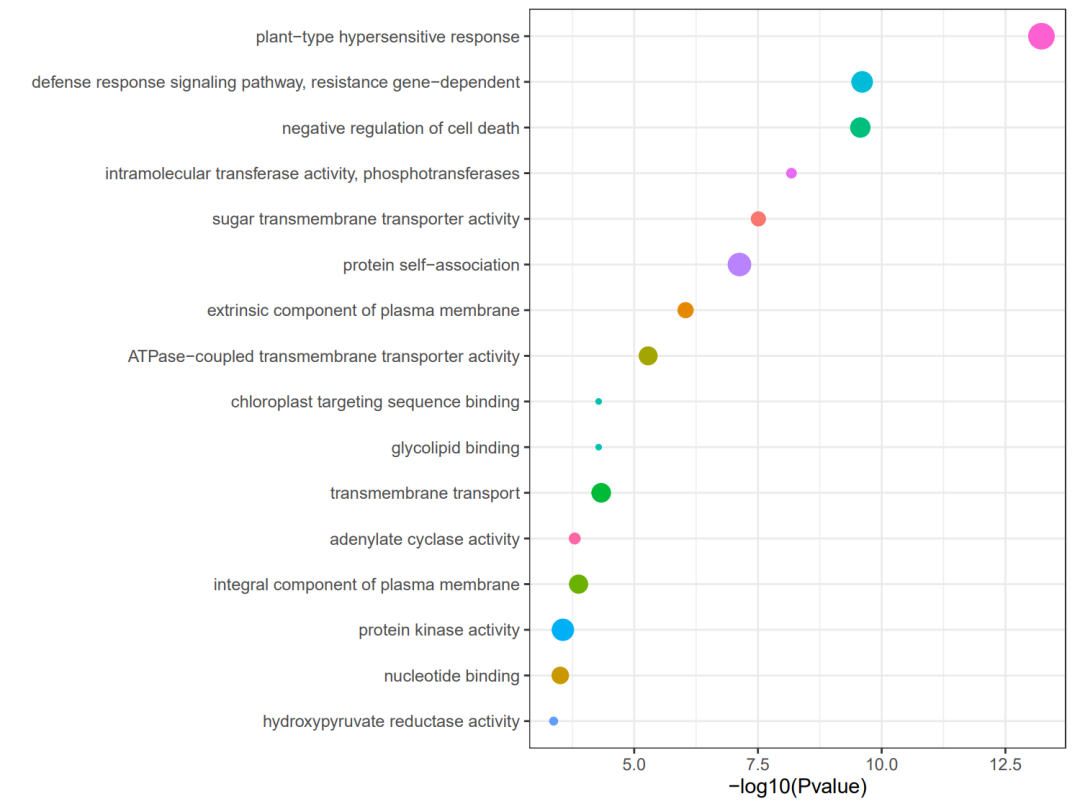+关注继续查看

# 速绘 丨 GO富集气泡图

## 前言介绍

• ID：表示具体的GO条目
• Description：GO条目的描述
• RatioF：分子是富集到这个GO条目上的gene的数目， 分母是所有输入的做富集分析的gene的数目
• RatioB：分母是所有编码蛋白的基因中有GO注释的gene的数目，分子是注释到这个GO条目上面的gene数目
• pvalue：富集的p值

• BP: biological process, 生物学过程
• MF: molecular function, 分子功能
• CC: cellular component, 细胞成分下面开始正式的绘图方法原理介绍

## 绘图方法

### 加载数据与参数

# 本脚本用于对TGT生成的GO结果作图
library(tidyverse)
rm(list=ls())
# 设置项目基本信息
file <- "GO-Table20230629105106.csv"  #数据文件名
job <- "20230629" #项目名称
out_info_num <- 30 #最大显示条目数
my_pvalue <- 1 #p值筛选条件
my_number <- 0 #数量筛选条件
color_1 <- c("blue","red") #颜色

df <- read.csv(file,header = T)
colnames(df) <- c("ID",
"Description",
"RatioF",
"RatioB",
"Pvalue",
"FDR",
"Number",
"Group",
"Gene")
df$Description <- factor(df$Description)
df <- df %>% filter(Pvalue < my_pvalue) %>%
filter(Number > my_number)

### 数据转换

df$RatioF <- mixedToFloat(df$RatioF)
df$RatioB <- mixedToFloat(df$RatioB)

df_plot <- df[order(df$RatioF),] leve_des <- unique(df_plot$Description)
df_plot$Description <- factor(df_plot$Description,levels = leve_des)
df_plot <- df_plot[1:out_info_num,]
df_plot <- df_plot %>% drop_na()

# 使用mutate函数替换Group列的值
df_plot <- df_plot %>%
mutate(Group = case_when(
Group == "molecular_function" ~ "MF",
Group == "cellular_component" ~ "CC",
Group == "biological_process" ~ "BP",
TRUE ~ Group
))

### 绘制图像

ggplot(df_plot)+
geom_point(aes(RatioF,Description,
color = Pvalue,
size = Number))+
labs(x="GeneRatio",y="GO description") +
labs(title="")+
theme_bw()+
facet_grid("Group",scales = "free_y",space = "free_y")
ggsave(str_c("Fig_GO_plot_",job,".pdf"),
width = 8,height = 8)

参考资料：
https://zhuanlan.zhihu.com/p/383654316
http://wheat.cau.edu.cn/TGT/m.html?navbar=GOEnrichment

“速绘”系列专注于分享便捷实用的作图脚本，低代码实现精美科研作图。主打的就是可重复使用，换套数据一行代码即可出图！本篇文章的绘图脚本已上传至后台，回复“GO29”即可获得。

|
1月前
|

r语言数据分析画数据相关性图热力图
r语言数据分析画数据相关性图热力图
27 1
|
4月前
|

R语言中如何进行PCA分析？利用ggplot和prcomp绘制基因表达量分析图（下）
R语言中如何进行PCA分析？利用ggplot和prcomp绘制基因表达量分析图（下）
57 0
|
4月前
R语言中如何进行PCA分析？利用ggplot和prcomp绘制基因表达量分析图（上）
R语言中如何进行PCA分析？利用ggplot和prcomp绘制基因表达量分析图
65 0
|
4月前
|

96 0
|
4月前
|

R语言数据可视化-气泡图

80 0
|
6月前
|
Go
ggplot2|绘制GO富集柱形图
ggplot2|绘制GO富集柱形图
121 0
|
6月前
|

GO-Figure! 是一个基于pyhton写的GO富集小软件，方便我们拿到富集结果后直接使用命令行的形式进行可视化，绘制出具有灵活性、可重复性的图形，且可基于最新的GO数据库内容进行富集。效果图如下，若用惯了常见的气泡柱形图，这种形式也不失为一种新颖的可视化方法~
101 1
|
6月前
|
Go 数据库 Python
Bingo(Cytoscape插件)GO功能富集分析

204 0
|
7月前
|

52 0
|
7月前
|

88 0